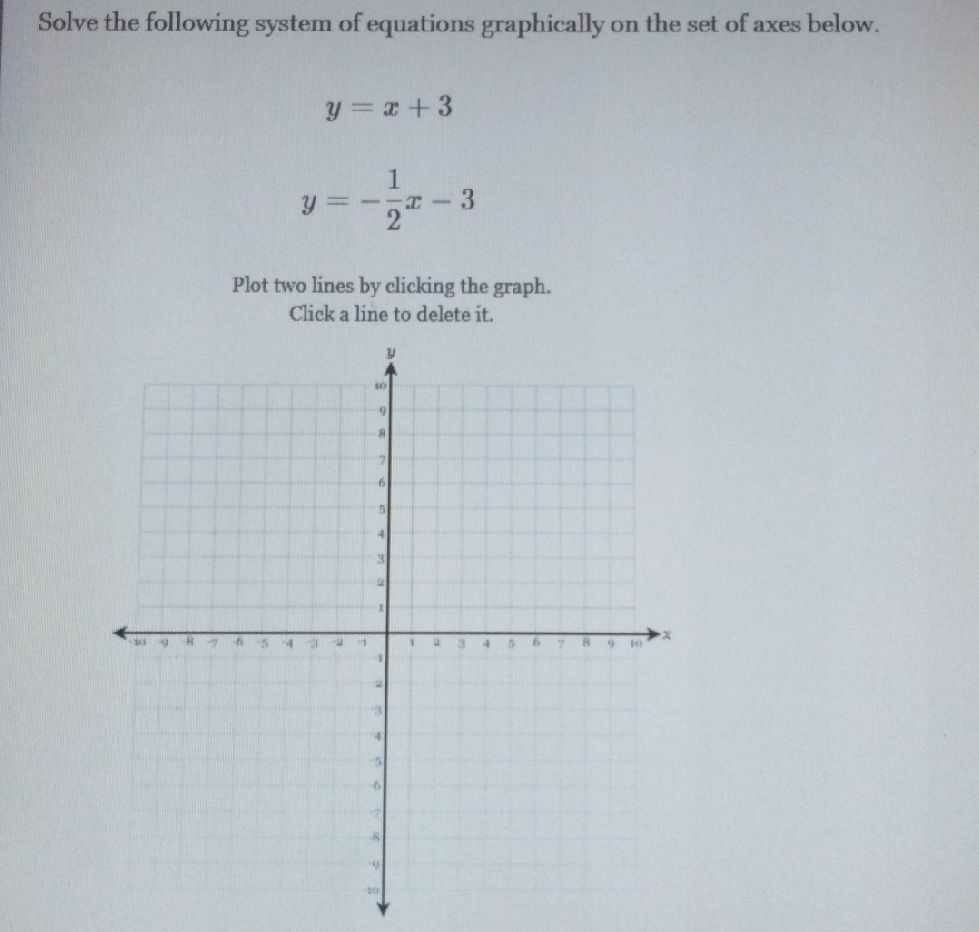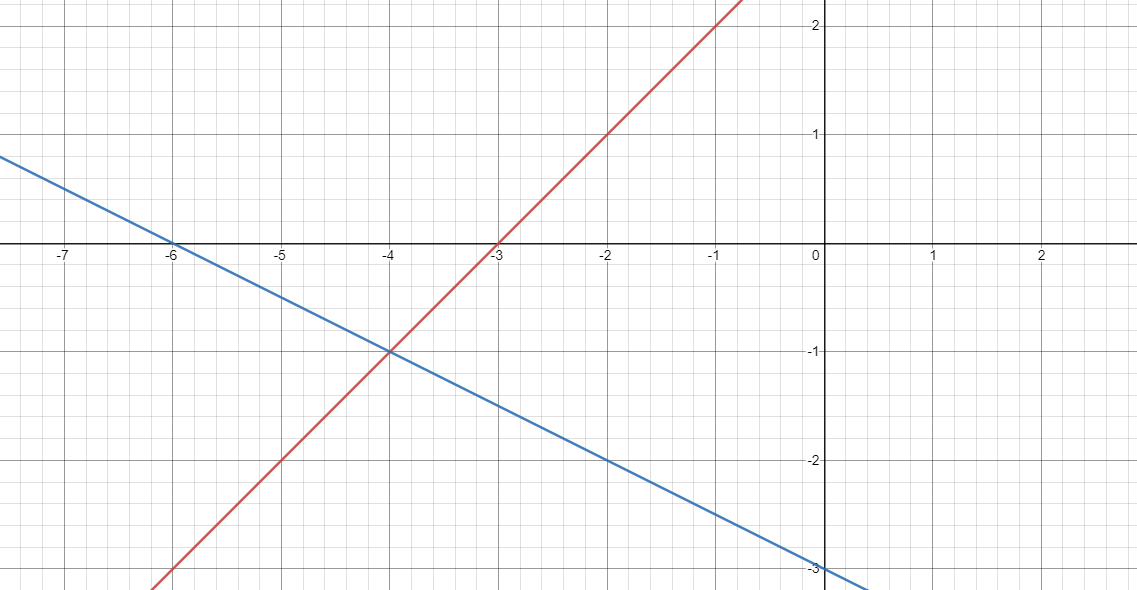### Still have math questions?Solve the following system of equations graphically on the set of axes below. $$y = x + 3$$$$y = - \frac { 1 } { 2 } x - 3$$Plot two lines by clicking the graph. Click a line to delete it.
(-4,-1)